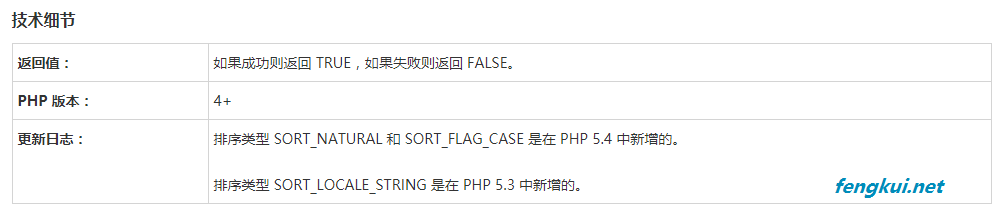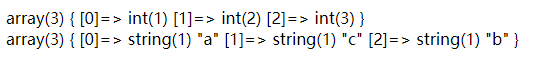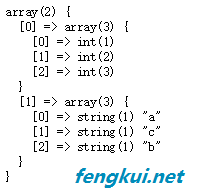# PHP根据键值对二维数组排序

``````/**
* [array_sort 多维数组排序]
* @param  [type] \$array [数组]
* @param  [type] \$key   [键名]
* @return [type]        [description]
*/
function array_sort(\$array, \$key) {
\$result = array();
\$values = array();
foreach (\$array as \$id => \$value) {
\$values[\$id] = \$value[\$key];
}
asort(\$values);
foreach (\$values as \$key => \$value) {
\$result[\$key] = \$array[\$key];
}
return \$result;
}
``````

``````/**
* [array_sort 多维数组排序]
* @param  [type] \$array [数组]
* @param  [type] \$key   [键名]
* @return [type]        [description]
*/
function array_sort(\$array, \$key, \$type='ASC') {
\$type = strtoupper(\$type) == 'ASC' ? SORT_DESC : SORT_ASC;
\$key_array = array_column(\$array, \$key);
array_multisort(\$key_array, \$type, \$array);
return \$array;
}
``````

### 说明：定义和用法

`array_multisort()` 函数返回一个排序数组。您可以输入一个或多个数组。函数先对第一个数组进行排序，接着是其他数组，如果两个或多个值相同，它将对下一个数组进行排序。
（当有多个数组时，每个数组都将根据前一个数组的顺序进行排序）``````\$a1 = array(2, 1, 3 );
\$a2 = array('c', 'a', 'b' );
array_multisort(\$a1, \$a2);
var_dump(\$a1); // array(3) { => int(1) => int(2) => int(3) }
var_dump(\$a2); // array(3) { => string(1) "a" => string(1) "c" => string(1) "b" }
``````这个例子里，排序后，第一个数组会包含 1、 2、 3。 第二个数组会包含 a、c、b。

``````\$array = array(
array(2, 1, 3 ),
array('c', 'a', 'b' )
);
array_multisort(\$array, SORT_ASC, \$array, SORT_DESC);
var_dump(\$array);
``````本例中，我们对第一个数组进行升序，对第二个数组降序，然后打印出总的数组，

``````\$array = array(
array('volume' => 67, 'edition' => 2),
array('volume' => 86, 'edition' => 1),
array('volume' => 85, 'edition' => 6),
array('volume' => 98, 'edition' => 2),
array('volume' => 86, 'edition' => 6),
array('volume' => 67, 'edition' => 7),
);

// 返回数组中指定的一列（array_column）
\$volume_array = array_column(\$array, 'volume');
\$edition_array = array_column(\$array, 'edition');

array_multisort(\$volume_array, SORT_DESC, \$edition_array, SORT_ASC, \$array);
var_dump(\$array);
``````